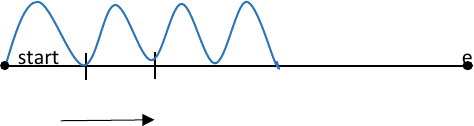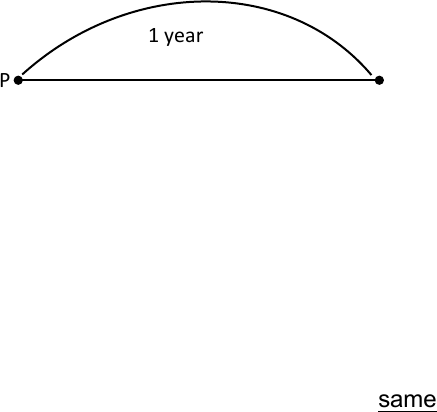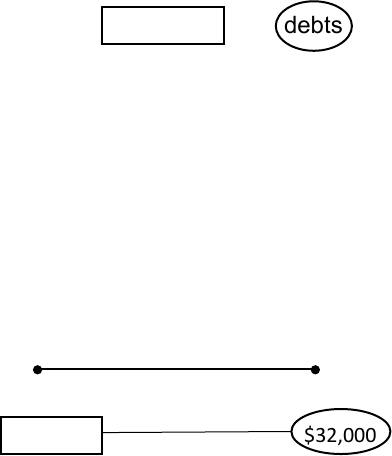Study Guides (390,000)
CA (150,000)
UTSC (10,000)
Mathematics (1,000)
MATA32H3 (100)
Midterm

# MATA32H3 Study Guide - Midterm Guide: Net Present Value, Binomial Theorem, FaradPremium

Department
Mathematics
Course Code
MATA32H3
Professor
Karimian Pour, C.
Study Guide
Midterm

This preview shows pages 1-2. to view the full 8 pages of the document.MATA32H3-Study Guide
Compound Interest Formula
S=P(1+r)^n
r = compound interest rate or periodic interest rate (amount of interest paid after a fixed
compound)
n = number of interest payments 1 2 3 4
S = compound amount
P = principle (amount invested) start end
Time
S=P(1+a/k)^kt
a = annual rate
k = compounded frequency/year
t = time or number of years
Example:
\$10,000 invested. 3.05% annual rate compounded monthly for 5 years.
P= \$10,000
a= 3.05% S=P(1+a/k)^kt
k= 12 S=10,000 (1+0.0305/12)^60
t= 5 = 11,645.17
S=11,645.17
*to ONLY find interest amount, take S-P
S-P
11,645.17-10,000
=1,645.17
Interest amount is 1,645.17

Only pages 1-2 are available for preview. Some parts have been intentionally blurred.

Subscribers OnlyEffective Rate of Interest
Suppose you invest P at r% APR compounding n times annually.
1 year
P S
P(1+r/n)^n
re is the effective rate of interest. re=(1+r/n)^n-1
The special rate of simple interest that yields the same amount as compounded interest.
*simple interest interest paid once a year (at the end of the year)
effective interest is also paid at the end of the year
re is bigger than r [binomial theorem]
when n is 1, ER and APR are the same
P(1+re) = P(1+r/n)^n
Example:
a=5% k=365 daily interest = 0.05/365
(1+0.05/365)^365 -1
= 0.0512657
= 5.12657%

Unlock to view full version

Subscribers Only

Only pages 1-2 are available for preview. Some parts have been intentionally blurred.

Subscribers OnlyEquation of Value
2 types: payments and debts
At all times, value of payment = value of debt
Example:
\$32,000 owed at 6 years \$10,000 paid
3.6 APR compounded quarterly
Find the balance payment
0 1 2 3 4 5 6
\$10,000 \$32,000
At t=6, the value of debt is \$32,000 and the value of payment is x.
t=6 k=24 a=0.036
\$20,035.86 owed